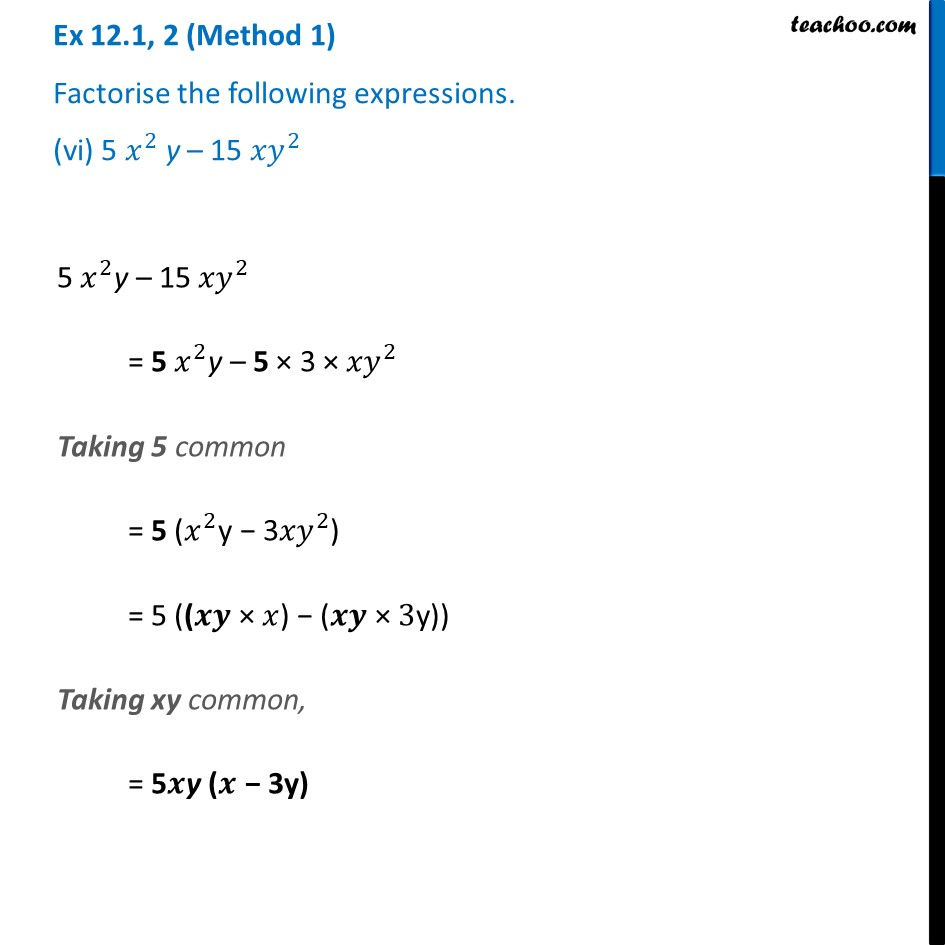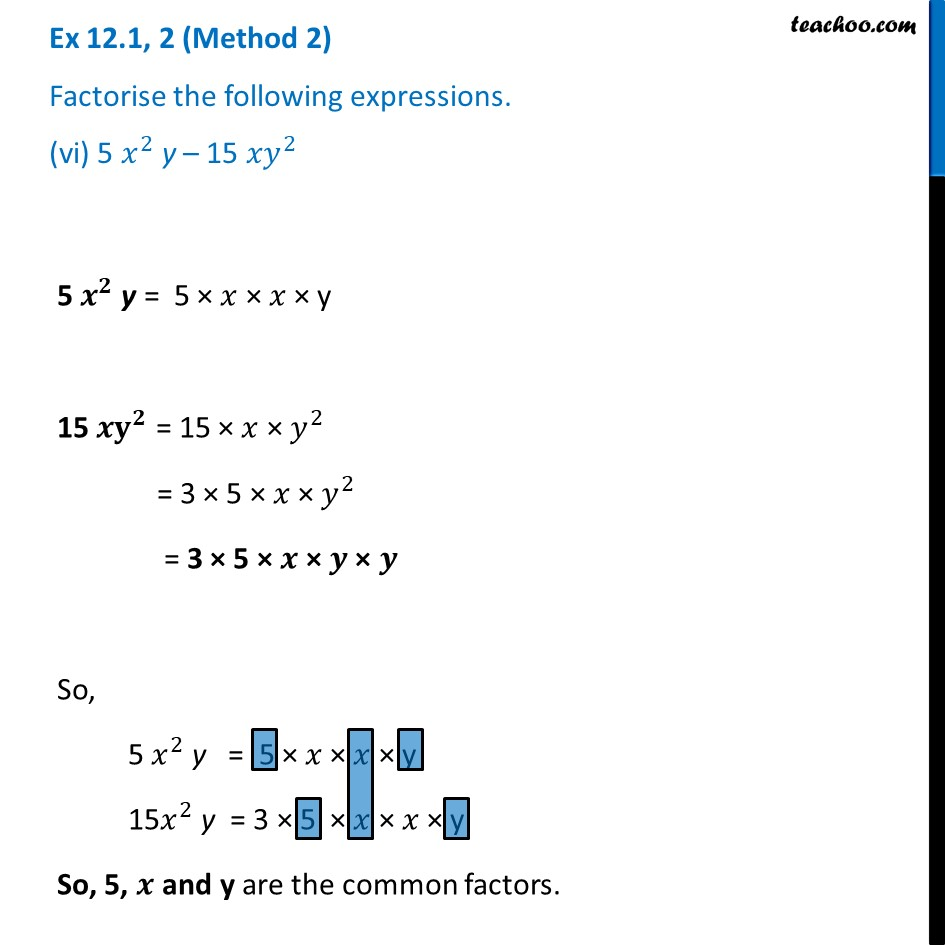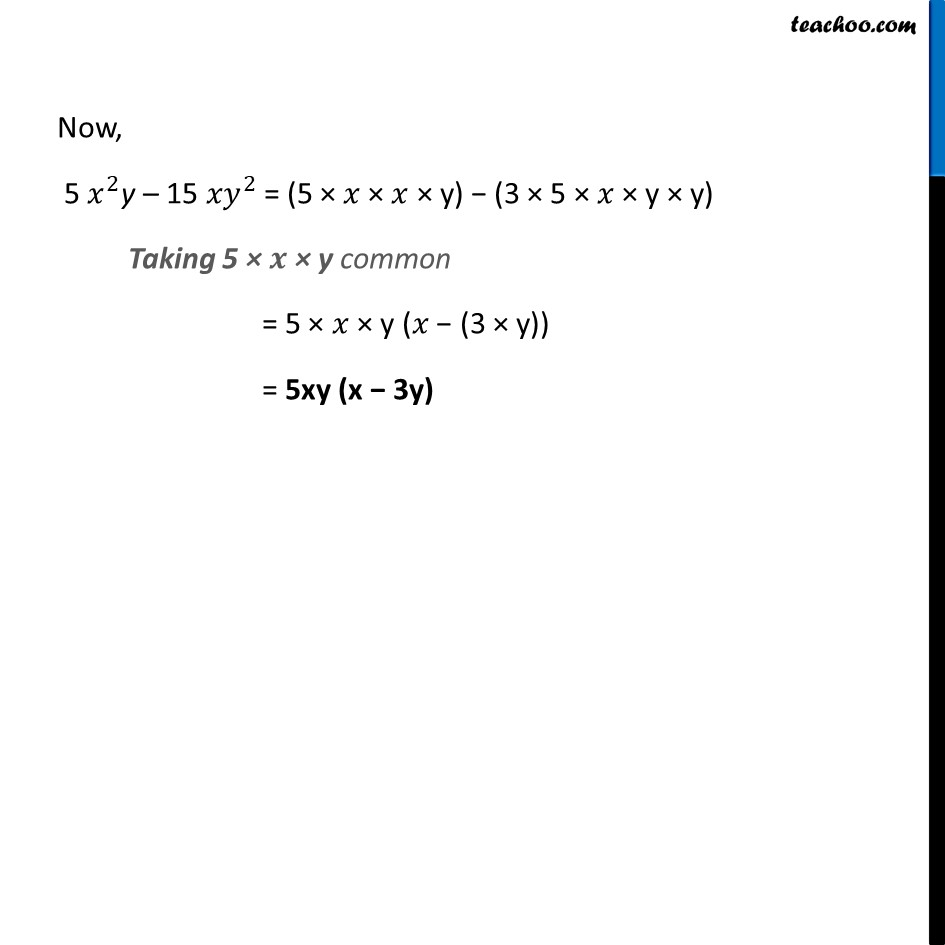Ex 12.1

Chapter 12 Class 8 Factorisation
Serial order wiseLearn in your speed, with individual attention - Teachoo Maths 1-on-1 Class

### Transcript

Ex 12.1, 2 (Method 1) Factorise the following expressions. (vi) 5 𝑥^2 y – 15 〖𝑥𝑦〗^25 𝑥^2y – 15 〖𝑥𝑦〗^2 = 5 𝑥^2y – 5 × 3 × 〖𝑥𝑦〗^2 Taking 5 common = 5 (𝑥^2y − 3〖𝑥𝑦〗^2) = 5 ((𝒙𝒚 × 𝑥) − (𝒙𝒚 × 3y)) Taking xy common, = 5𝒙y (𝒙 − 3y) Ex 12.1, 2 (Method 2) Factorise the following expressions. (vi) 5 𝑥^2 y – 15 〖𝑥𝑦〗^25 𝒙^𝟐 y = 5 × 𝑥 × 𝑥 × y 15 𝒙𝐲^𝟐 = 15 × 𝑥 × 𝑦^2 = 3 × 5 × 𝑥 × 𝑦^2 = 3 × 5 × 𝒙 × 𝒚 × 𝒚 So, 5 𝑥^2 y = 5 × 𝑥 × 𝑥 × y 15𝑥^2 y = 3 × 5 × 𝑥 × 𝑥 × y So, 5, 𝒙 and y are the common factors. Now, 5 𝑥^2y – 15 〖𝑥𝑦〗^2 = (5 × 𝑥 × 𝑥 × y) − (3 × 5 × 𝑥 × y × y) Taking 5 × 𝒙 × y common = 5 × 𝑥 × y (𝑥 − (3 × y)) = 5xy (x − 3y)